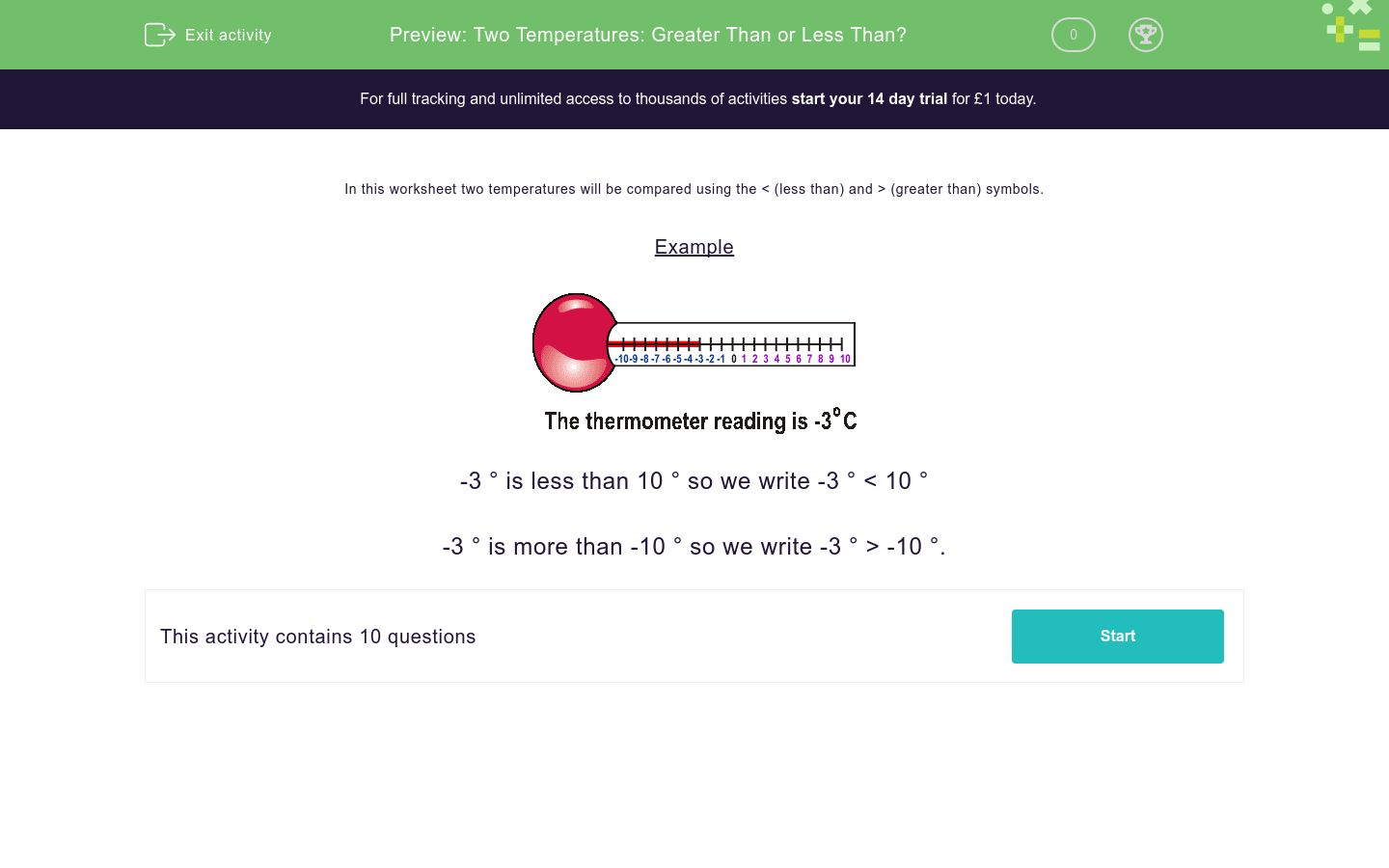# Two Temperatures: Greater Than or Less Than?

In this worksheet, students look at a number (which may be negative) and decide whether the number that follows it is greater than (>) or less than (Key stage:  KS 2

Curriculum topic:   Number: Number and Place Value

Curriculum subtopic:   Recognise Thousands, Hundreds, Tens and Ones

Difficulty level:### QUESTION 1 of 10

In this worksheet two temperatures will be compared using the < (less than) and > (greater than) symbols.

Example-3 ° is less than 10 ° so we write -3 ° < 10 °

-3 ° is more than -10 ° so we write -3 ° > -10 °.

Put the right symbol > or < between the two numbers.

0 ° -9 °
<

>

Put the right symbol > or < between the two numbers.

-5° 9 °
<

>

Put the right symbol > or < between the two numbers.

-3° -7°
<

>

Put the right symbol > or < between the two numbers.

-3° -10°
<

>

Put the right symbol > or < between the two numbers.

-9° -5°
<

>

Put the right symbol > or < between the two numbers.

<

>

Put the right symbol > or < between the two numbers.

-10°

<

>

Put the right symbol > or < between the two numbers.

-9°
<

>

Put the right symbol > or < between the two numbers.

-7°
<

>

Put the right symbol > or < between the two numbers.

-8°  6°
<

>

• Question 1

Put the right symbol > or < between the two numbers.

0 ° -9 °
>
EDDIE SAYS
On the number line, 0 > -9.
• Question 2

Put the right symbol > or < between the two numbers.

-5° 9 °
<
EDDIE SAYS
On the number line, -5 < 9.
• Question 3

Put the right symbol > or < between the two numbers.

-3° -7°
>
EDDIE SAYS
On the number line, -3 > -7.
• Question 4

Put the right symbol > or < between the two numbers.

-3° -10°
>
EDDIE SAYS
On the number line, -3 > -10.
• Question 5

Put the right symbol > or < between the two numbers.

-9° -5°
<
EDDIE SAYS
On the number line, -9 < -5.
• Question 6

Put the right symbol > or < between the two numbers.

>
EDDIE SAYS
On the number line 8 > 1.
• Question 7

Put the right symbol > or < between the two numbers.

-10°

<
EDDIE SAYS
On the number line, -10 < 4.
• Question 8

Put the right symbol > or < between the two numbers.

-9°
<
EDDIE SAYS
On the number line, -9 < 8.
• Question 9

Put the right symbol > or < between the two numbers.

-7°
>
EDDIE SAYS
On the number line, 7 > -7.
• Question 10

Put the right symbol > or < between the two numbers.

-8°  6°
<
EDDIE SAYS
On the number line, -8 < 6.
---- OR ----

Sign up for a £1 trial so you can track and measure your child's progress on this activity.

### What is EdPlace?

We're your National Curriculum aligned online education content provider helping each child succeed in English, maths and science from year 1 to GCSE. With an EdPlace account you’ll be able to track and measure progress, helping each child achieve their best. We build confidence and attainment by personalising each child’s learning at a level that suits them.

Get started